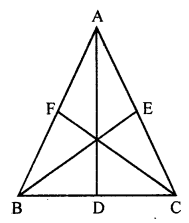## ML Aggarwal Class 7 Solutions for ICSE Maths Chapter 11 Triangles and its Properties Ex 11.1

Question 1.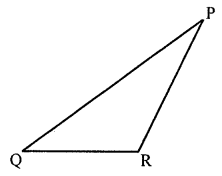(i) Name the vertex opposite to side PQ.
(ii) Name the side opposite vertex Q.
(iii) Name the angle opposite to side QR.
(iv) Name the side opposite to ∠R.
Solution:
In the given figure,
(i) Vertex opposite to side PQ is R.
(ii) The side opposite to the vertex Q is PR.
(iii) Angle opposite to the side QR is ∠R.
(iv) the side opposite to ∠R is PQ.

Question 2.
Look at the figures given below and classify each of the triangles according to its (a) sides (b) angles
(You may judge the nature of the angle by observation):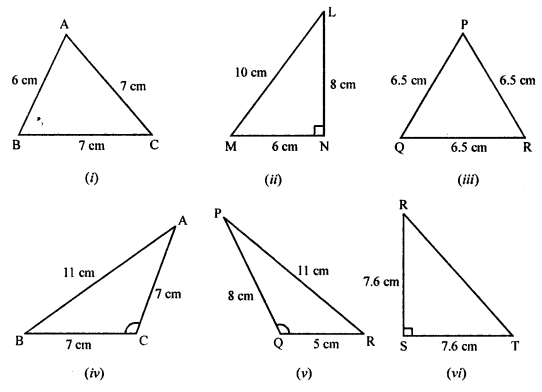Solution:
(i) Two sides are equal. So it is an isosceles triangle.
(ii) Three sides are unequal and one angle is 90°,
so it is a right-angled triangle end also it is a scalene triangle also.
(iii) Three sides are equal. So, it is an equilateral triangle.
(iv) Two sides are equal. So, it is an isosceles triangle.
It’s one angle is obtuse, therefore it obtuse angled triangle also.
(v) Three sides are not equal. So, it is a scalene triangle.
It’s one angle is obtuse. So, it is an obtuse angled triangle also.
(vi) Its two sides are equal and one angle is a right angle.
So it is a right-angled isosceles triangle.

Question 3.
In the given ∆PQR, if D is the mid-point of $$\bar { QR }$$, then
(i) $$\bar { PM }$$ is ……..
(ii) $$\bar { PD }$$ is ………
Is QM = MR?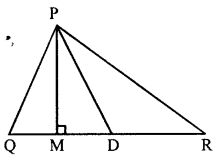Solution:
If the given figure, in ∆PQR
D is mid-point of $$\bar { QR }$$, then
(i) PM is an altitude.
(ii) PQ is the median.
No, QM ≠ MR

Question 4.
Will an altitude always lie in the interior of a triangle? If no, draw a rough sketch to show such a case.
Solution:
No, it is not necessary, it may lie outside the triangle also.
Here is given a rough sketch of the case AD is the altitude of ∆ABC.
Draw from A to the side CB (produced).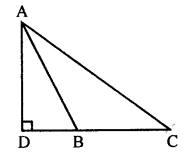Question 5.
Can you think of a triangle in which two altitudes of the triangle is its sides? If yes, draw a rough sketch to show such a case.
Solution:
Yes, it is a right-angled triangle.
Here, AB ⊥ BC and BC ⊥ AB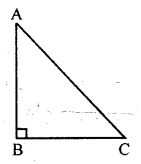Question 6.
Draw rough sketches for the following:
(i) In ∆ABC, BE is a median of the triangle.
(ii) In ∆PQR, PQ and PR are altitudes of the triangle.
(iii) In ∆XYZ, YL is an altitude in the exterior of the triangle.
Solution:
(i) In ∆ABC, BE is the median of the triangle.
E is the mid-point of AC. So, BE is the median.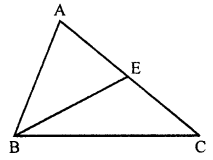(ii) In ∆PQR, PQ and PR are the altitudes of the triangle.
In ∆PQR, ∠P = 90°
So, PQ and PR are the altitudes.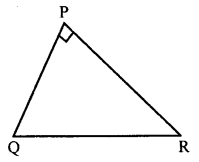(iii) In ∆XYZ, YL is the altitude in the exterior of the
triangle YL is altitude on ZX (produced).Question 7.
Take an equilateral triangle and draw its medians and altitudes and check that the medians and altitudes are the same.
Solution:
∆ABC is an equilateral triangle.
AD, BE and CF are altitudes of the triangle.
The altitudes of an equilateral triangle
divide the sides into two equal parts.
So, altitudes are also the medians of the triangle.